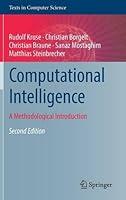# Computational Intelligence: A Methodological Introduction, 2nd Edition## Book Description

This textbook provides a clear and logical introduction to the field, covering the fundamental concepts, algorithms and practical implementations behind efforts to develop that exhibit intelligent behavior in complex environments. This enhanced second edition has been fully revised and expanded with new content on swarm intelligence, deep learning, fuzzy data analysis, and discrete decision . Features: provides supplementary material at an associated website; contains numerous classroom-tested examples and definitions throughout the text; presents useful insights into all that is necessary for the successful application of computational intelligence methods; explains the theoretical background underpinning proposed solutions to common problems; discusses in great detail the classical areas of artificial neural , fuzzy and evolutionary algorithms; reviews the latest developments in the field, covering such topics as ant colony optimization and probabilistic graphical .

Chapter 1 Introduction to Computational Intelligence

Part 1 Neural Networks
Chapter 2 Introduction to Neural Networks
Chapter 3 Threshold
Chapter 4 General Neural Networks
Chapter 5 Multilayer Perceptrons
Chapter 6 Radial Basis Function Networks
Chapter 7 Self-organizing Maps
Chapter 8 Hopfield Networks
Chapter 9 Recurrent Networks
Chapter 10 Mathematical Remarks for Neural Networks

Part 2 Evolutionary Algorithms
Chapter 11 Introduction to Evolutionary Algorithms
Chapter 12 Elements of Evolutionary Algorithms
Chapter 13 Fundamental Evolutionary Algorithms
Chapter 14 Computational Swarm Intelligence

Part 3 Fuzzy Systems
Chapter 15 Introduction to Fuzzy Sets and Fuzzy Logic
Chapter 16 The Extension Principle
Chapter 17 Fuzzy Relations
Chapter 18 Similarity Relations
Chapter 19 Fuzzy Control
Chapter 20 Fuzzy Data Analysis

Part 4 Bayes and Markov Networks
Chapter 21 Introduction to Bayes Networks
Chapter 22 Elements of Probability and Graph Theory
Chapter 23 Decompositions
Chapter 24 Evidence Propagation
Chapter 25 Learning Graphical Models
Chapter 26 Belief Revision
Chapter 27 Decision Graphs